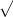Melayah M.

# I need help with this question Calculus

Apply Newton's Method to approximate the x-value(s) of the indicated point(s) of intersection of the two graphs. Continue the iterations until two successive approximations differ by less than 0.001. [Hint: Let h(x) = f(x) − g(x).]

f(x) = 2x + 2

 g(x) =x + 6

By: